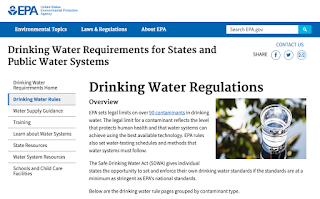## Wednesday, January 20, 2021

### Parts Per Million

General Chemistry Index

Where are we going with this? This page will assist in developing the ability to perform calculations to determine the composition of a compound or mixture when given the necessary information and to apply lab data to determine the empirical and molecular formula of a compound.

Parts Per Million
This doesn't sound bad, does it?https://www.epa.gov/dwreginfo/drinking-water-regulations

You might have heard of his one. Like, how many parts of something is allowed in something.

The definition is pretty obvious… Parts per million is how many times (by mass) that something appears within a million parts of the whole thing. It is abbreviated as PPM.

A more formal definition:

Parts Per Million (ppm) is the number of units of mass of a substance per million units of total mass.

Because of the relationship between grams and water, it turns out that PPM in water turns out to be:

PPM = mg / l

where mg is mass of the "added in thing" and l is volume of water in liters.

PPM is often used to discuss contaminants in something. For instance, EPA states that drinking water needs to not have more than 1.3 parts per million of copper in it. Most utility agencies are required to report how their water tests, and often it will include PPM data.

Besides PPM, concentrations can be reported at even lower concentrations. PPB is parts per billion. Both are analogous to percent, witch is parts per one hundred.2021-01-21 Air Quality,Lowell, Indiana

Air Quality is also discussed in terms of what part of the whole contains contaminants. The amounts present are often reported in PPM or PPB.

Okay, that's nice… Hmm… Seems like there would be some math here, right?

So, yeah… Math…

Let's think about this… You know… logic… If something is half and half, it would be 500,000 parts per million, right?

So, if you take the mass of the part divided by the mass of the whole, then multiply by 1,000,000, you'll have PPM, right?

Let's try!

Mass of whole = 1,000,000
Mass of part = 500,000

Part / Whole • 1,000,000 = PPM

500,000 / 1,000,000 • 1,000,00 = PPM
.5 • 1,000,000 = PPM
500,000 = PPM

Well, logic and formula converged nicely! That's always a good thing!https://sciencing.com/calculate-concentration-ppm-6935286.html
So, let's say that the formulas for PPM are…

PPM = Mass of Part / Mass of Whole • 1,000,000

Mass of Part = PPM * Mass of Whole /1,000,000

Mass of Whole = Mass of Part • 1,000,000 / PPM

because algebra…

Let's keep going! Let's say that ThingX has a concentration of 2 parts per million in a mixture with ThingY.

So, if you have 8 million grams of ThingY, what is the mass of ThingX?

Mass of Whole • PPM /1,000,000 = Mass of Part

8,000,000 gr • 2 /1,000,00 = Mass of Part
16 gr = Mass of Part

_____________________________
SO WHAT?